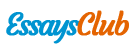Autor:   •  January 13, 2019  •  3,100 Words (13 Pages)  •  196 Views

Page 1 of 13

...

3.2.5 Items counted table

Figure 6

Figure 6 above shows the items counted from the suppliers. The numbers of items which are less than or equal to 10, the results was 5 because form the cell B12 to the cell H15 there were only 5 items which were less than \$10. The number of items that user spends more than \$200; the result was zero because from cell B12 to cell B15 there was no number which is more than \$200, The formulas used are shown below:

3.3 Flower stalks gallery worksheet

This worksheet contains two tables which are: Flower types table and Calculations on flower types table.

3.3.1Flower types table

Figure 7

Figure 7 above shows different types of flower types including blue stalks, pink stalks, purple stalks, red stalks, white stalks and yellow stalks with reference to the amount in rose, carnation and statices.

3.3.2 Calculation on flower types table

Figure 8

Figure 8 above shows the calculations on the types of flower which include blue stalks, pink stalks, purple stalks, red stalks, white stalks and yellow stalks.

The total number of stalks is calculated to show the total amount of rose, carnation and statice and the INT () function was used; Number of free stalks was calculated to show that if the customer exceeds the minimal order they will get free stalks. The price is calculated from the supplier worksheet where the sum of number of stalk is divided by the number of one dozen and multiplied by the price of the particular price of that item. Remarks were placed in the table to let the user know if he reach the minimal criteria to get the free stalk or not, if the customer reach the minimal order to get the free stalk it will show enough and if not it will tell the customer the minimal order which is needed. The total price is calculated from the row containing price. And the formulas are shown below:

Total number of stalks: Rose Carnation Statice

Number of free stalks: Rose Carnation

This worksheet shows contains two tables which are Accessories supply table and calculations on accessories supply table.

3.4.1 Accessories supply table

Figure 9

The figure 9 above shows the accessories types which include plastic wraps, basket, ribbons and cards. In this table the price is calculated from the supplier worksheet where the sum of number of stalk is divided by the number of one dozen and multiplied by the price of the particular price of that item. The tax charge was calculated using absolute reference , by multiplying the price and the 5% which is fixed. The total accessories price is calculated by adding the price and the tax charges.

Price Tax charger

3.4.2 Calculations on accessories supply table

Figure 10

The Figure 10 above shows the calculations on tax which is done by absolute reference cell, sub-total accessories price, delivery and total price. Sub-total accessories price is calculated by adding the entire total accessories price. Delivery is calculated by providing criteria which is, it should include 5% delivery only when the sub-total accessories price is less than \$1000 if it is more than \$1000 then the delivery should be zero. The Total price is calculated by adding up the sub-total accessories price and the delivery.

3.5 Sweet factory worksheet

In this worksheet there are two tables which are sweet items table and the table on the calculations on sweet items.

3.5.1Sweet items table

Figure 11

Figure 11 above shows the items including chocolate, chocolate-chip cookies and candies. The price is calculated by multiplying the cell containing the package quantity and the price of the items from the supplier worksheet. Price After discount is calculated using the criteria that if the price is greater than or equal to \$500 the discount should be 3% of the price, if the price is greater than or equal to \$800 the discount should be 4% of the price and if the price is greater than or equal to \$1000 the discount should be 5% of the price.

3.5.2 Calculations on sweet items table

Figure 12

The figure 12 above shows calculations which are sub-total after discount, delivery and total price. The sub-total a. discount is calculated by adding up the price after discount. The total price is calculated by adding the sub-total price after discount and the delivery which is \$10.

Sub-total price after discount Total price

3.6 Chart worksheet

This worksheet contains four bar charts present the prices for the suppliers which are flower stalks gallery chart, gift accessories supply chart, sweet factory chart and comparison chart.

3.6.1 Flower stalks gallery chart

Figure 13

Figure 13 above represent the spending analysis for flower

...